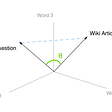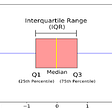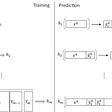# Generalized Linear Models (GLMs)

In this article, I would like to discuss some behind the scene works of linear regression and logistic regression as two introductory machine learning algorithms. Particularly, the reason we simply call them linear models and how their origins are tied together.

For this, we start with the simple recurring regression example of housing prices. Suppose we wish to predict the price tag of houses in a certain region based on the size. The first step is to collect our data. We knock on each door and acquire the square footage and the price from the occupants. Note that there might exist several houses of the same size x with different prices. Which price should we predict for size x then? well, intuitively we would like to be able to predict a price that makes the most sense; one that in a way is the mean or median of these prices.

More formally, let’s assume that the collected prices for size x are distributed around an average value with a certain degree of variation. In other words, prices are distributed according to a Gaussian distribution. This is not a far-fetched assumption as most data in our world is Gaussian. In this scenario, people are not going to be too greedy to propose an outrageously expensive price. Hopefully, they have a good idea of what a house of that size is worth in that region and will only nudge the amount up a bit due to a financial situation or lower the price a tad to meet their deadline to sell the property and move out:

It helps to visualize this distribution on the size-price graph as such:

The point of these graphs is to convey that at each point on the x-axis, we would like our model to predict the most likely price, i.e. the expected value of the gaussian distribution. So that next time someone asks for the price of a say 6800 sqft house, we don’t give them the most expensive price tag or the cheapest deal that you’d have to be lucky Luke to get, but the most reasonable price-tag that a house this size usually goes for. And since the expected value of a Gaussian distribution is its mean in the middle, the regression line would go through the means of these distributions like below:

We would solve this regression problem via maximum likelihood estimation (refer to this for a quick refresher) which would eventually lead to the following update rule for parameters :

in which function h(x) which is our model, is equal to:

Now, this was one special case where the distributions at each point x were Gaussian. We will undoubtedly run into problems where the distribution is Bernoulli, Poisson, Gamma, etc. The general procedure is the same; maximum likelihood estimation. It turns out, there is a group of distribution for which the steps to solve the problem are meaningfully similar. For example, Solving the same problem for the Bernoulli distribution (binary classification) leads to the minimization of the following cost function:

in which the model h(x) would be:

Note that although h(x) for this binary case is not the same as the Gaussian case, the only difference is that h(x) in the Gaussian case goes through another function namely sigmoid, to become appropriate for the Bernoulli distribution:

People realized that his pattern recurs for Poisson, Gamma, and a number of other distributions as well. The update rule always ends up being:

Where h(x) is basically a post-processed version of the Gaussian h(x) gone through another function g to make it appropriate for the corresponding distribution. This function g is referred to as the canonical response function. Previously for the Bernoulli distribution, the sigmoid function was pulled out of thin air to express g. The GLM procedure enables us to derive the canonical response function.

So these observations can intrigue us to look for a more generalized strategy to find a model for this family of distributions and avoid blindly repeating the same process for each distribution separately. This helps because if there is a new problem with a new distribution we have never encountered before but can verify that it belongs to the same family, we can simply apply the strategy to find our model (hypothesis h(x)), the cost function we need to minimize, etc.

So let’s learn about this family. What distributions does it include? how can we verify whether a certain probability belongs to this family or not?

# Exponential Family Distribution

Exponential family distributions are probability distributions that can be arranged in the following format:

This is the family of distributions we have been talking about all along. In this notation, η is called the natural parameter (canonical parameter) for the distribution. T(y) is called the sufficient statistic, b(y) is called the “base measure”, and a(η) is referred to as the log partition function. The term η^ T (forgive the crummy notation) is actually the transpose of η and has nothing to do with T(y). It is worth noting that usually η ends up being a scalar and T(y)=y for the most part.

Exponential family distributions have some nice properties such as:

And the fact that log(p; η) is concave and therefore we are sure to find a local maximum for through our maximum likelihood estimation approach. This information is helpful, however, we won’t take the long road of MLE to find models for these distributions.

A set of b(y), T(y), and a(η) determines a certain member from the family parametrized by η, and changing this parameter η yields a specific distribution from this member. For example, we will see shortly that a set of b(y), T(y), and a(η) results in the Gaussian distribution formula in which altering the value of η would be equivalent to altering the mean of the Gaussian distribution.

Now pick your probability distribution of interest, try and see if you can cosmetically shape it into the above equation and if you could, you've got yourself a distribution from the exponential family. Let’s illustrate why Gaussian distribution belongs to this family. We know the equation for Gaussian distribution as (For simplicity, variance is assumed to be 1 here. However, you can include the variance parameter in the equation for practice):

We can rewrite this to be:

Therefore if we match this equation to that of the Exponential family, with the following assignments Gaussian distribution can be assumed an Exponential family member:

This is enough to prove that Gaussian distributions are a member of the Exponential family. Let’s take Bernoulli distribution as another example. We know the equation for Bernoulli to be:

Rearranging the above equation we can write:

So with the following set of assignments Bernoulli distribution is a member of the Exponential family:

Similarly, any other distribution’s membership can be verified based on whether it can be written in the general Exponential family format.

# Modeling for Exponential family

Linear regression and logistic regression are two special cases of Generalized Linear Models. These are models driven for Exponential family distributions and since they all fall under the same category, there is a general strategy to derive them.

Suppose that the distribution you are working on to model is an exponential family member. The following two assumptions spare us the maximum likelihood procedure or any guesswork about what the function h(x) should be to properly model the problem.

1. Our goal is for the model to predict the expected value of at each point (h(x) = E[y|x]). For instance, as discussed in the previous section, we would like to predict the most reasonable price for a house of size 6800sqft rather than an improbable cheap or expensive deal.

2. As a design choice, we set η= θx as a way to relate the two distribution parameters η and θ so we can translate E[y|x; η] to E[y|x; θ] and vice versa.

To reiterate, the overall idea is to find out whether the distribution of data can be expressed as a member of the Exponential family. If that is the case, following the two assumptions above will tender the model for prediction.

As an example, let’s derive the linear regression model with this approach. We assumed that our data for regression is Gaussian therefore the first step would be to verify Gaussian distribution is a member of the Exponential family which we have done above with the following assignments:

According to (1), we are supposed to estimate E[y|x; θ]. Therefore:

In the above, we used the fact that the expected value of a Gaussian distribution Ν(μ, σ²) is equal to its mean μ.

For another example, let’s derive logistic regression. We know the data (probability of a y conditioned on x) to be Bernoulli and Bernoulli is shown above to be an Exponential family member with these assignments:

So we can simply apply our GLM strategy and derive:

Note that here sigmoid function is derived to represent the canonical response function. In the same manner, prediction models (or rather canonical response function g) for Poisson, Gamma, and other distributions will simply be rendered as a product of this approach.

1. It was mentioned that our goal is to predict E[y|x] at each point but actually, we would predict the expected value of T(y), however, since it usually equals y and it is more intuitive to use y I chose not to mention anything. It feels like TMI.

There you have it, GLMs. We went over Exponential family distributions, generalized linear models, and how to exploit this method to make predictions for a variety of distributions.

In the end, I would like to thank CS229 of Stanford University for graciously open-sourcing a generous portion of their material.

--

--

--

## More from Majid

Love podcasts or audiobooks? Learn on the go with our new app.

## Deep Learning — Technology behind Artificial Intelligence## How to run Neural Network on STM32 (Part 3)## The Hidden Dangers of Language Models## Train your Deep Neural Network in Azure VM## Lifespan Age Transformation Synthesis## Introduction to Artificial Neural Network## AI and Machine Learning in Quant Trading… Is it all hype? Is it a revolution? What are some issues?## Types of Transformations in Machine Learning## Recognizing Handwritten Digits with Scikit-learn